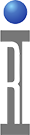Noise Figure Measurements

Definitions
What is Noise Figure
Y Factor Type Measurements
Second Stage Error Correction
Mismatch Effects
System Noise Figure Effects

The Cassini and RI 7100A Microwave ATE Systems perform high performance noise figure measurements using the loss calibration factors determined with the S-parameter measurement hardware previously discussed and the built-in electronic noise source in the RF Test Head for a traditional Hot/Cold Noise Figure Measurement. The following slides will review the definition of noise figure, describe the modified Y factor measurement process, discuss the importance of second stage noise correction, mismatch errors and discuss how the RI ATE System performs noise figure measurements.

Available Noise Power (Thermal)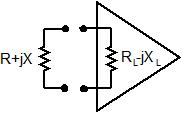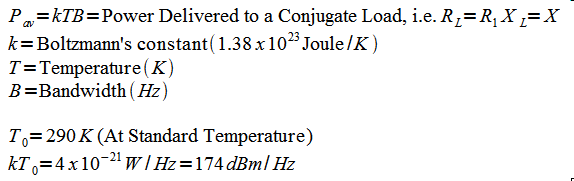Definition

Before we define noise figure we need to first define thermal noise power available from a passive device such as a resistor using the theoretical work performed by Nyquist and Johnson. The thermal noise power delivered to a conjugate load at temperature T and In a Bandwidth B is equal to kTB where k is Boltzmann’s constant.

Noise Figure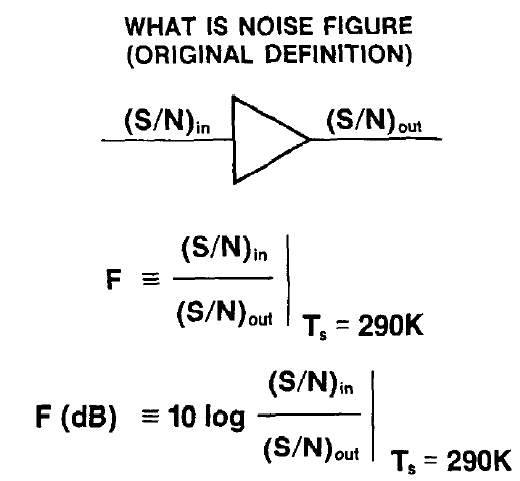Definition

The noise figure of a two port device (a device with two RF ports) is a measure of how the thermal noise and the shot noise generated in a device degrades the S/N (signal to noise ratio) from the input to the output of the device. This definition for noise figure applies to both the linear and log units.

Noise Figure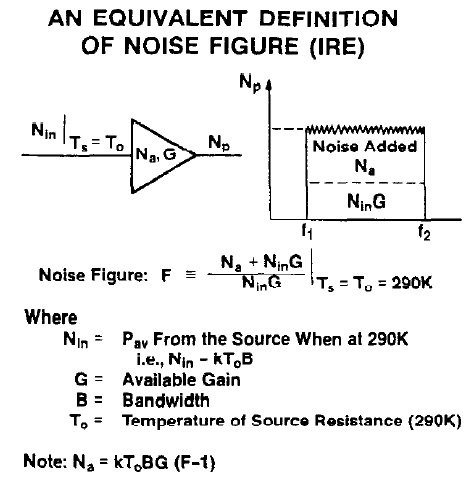Another Definition

Since Signal to Noise ratio is difficult to measure directly, we can define the Noise Figure an alternative way, but we need to understand the additive properties of uncorrelated noise. The noise power at the output of a two-port device is the sum of the noise present at the device’s Input (Nin) which is amplified by the device’s gain (G), plus the noise contributed by the device (Na). Specifically Nout=Nin x G + Na. If we substitute Nout into the equation from the previous slide and solve for F again we obtain the equation shown above.

Noise Figure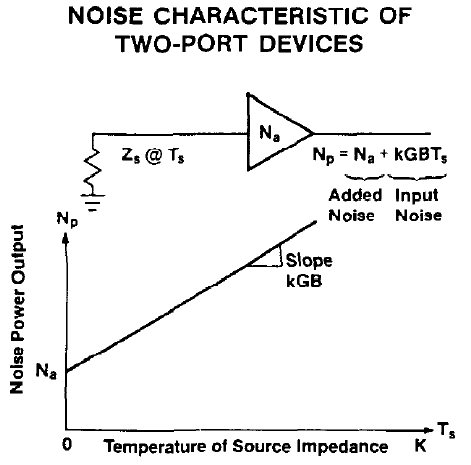Two Port Devices

If we terminate the input of a two port device with a load set at a temperature Ts, the noise power present using our original equation would be Nin=K x Ts x B. We see that the noise power present in a bandwidth B is a linear function of the temperature of the source impedance Zs. Note that if the temperature of the load (Ts) is equal to 0 degrees K then the noise power at the output of the device (Np) would be equal to the noise power contributed by the device (Na).

Noise Figure Measurements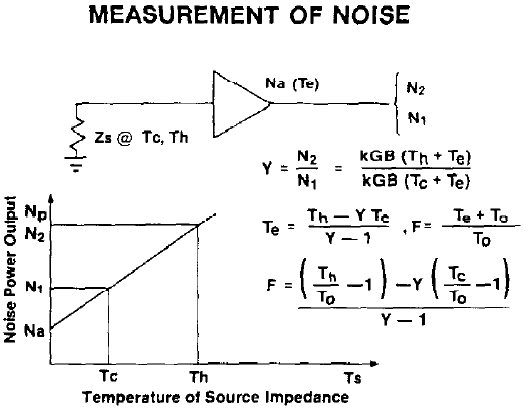Y Factor Noise Figure Measurements

Y factor noise figure measurements are the most common method for determining a device’s noise figure and the standard approach used by the Cassini and RI 7100A ATE systems. This approach requires applying two known levels of thermal noise (k x Th x B & k x Tc x B) to the input of the DUT and measuring the resulting levels of output noise power (N2 & N1) in a known measurement bandwidth, B. The two noise levels are generated by turning on (k x Th x B) and off (k x Tc x B) an electronic noise source. These two noise levels are referred as the hot (noise source on) and cold (noise source off) noise levels, respectively. Th and Tc are referred to as the hot and cold noise temperatures, respectively. The ratio of the two resulting output noise levels N2/N1 Is referred to as the Y factor. The noise figure (F) and the effective input noise (Te) can be calculated using the equations shown.

Second Stage Noise Contribution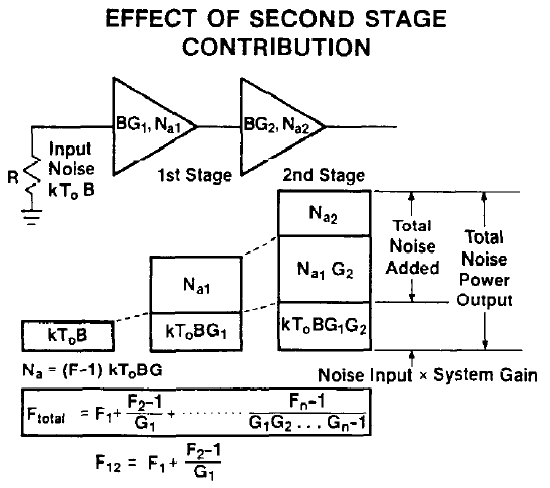Calculating the Second Stage Noise Contribution

The equation for calculating the total noise figure (Ftotal) for a series of cascaded devices is shown above. This equation is called the cascade noise equation. This equation consists of a series of linear terms. Each term represents the noise contribution for each stage of the network. As you can see the contribution to the total noise figure of each succeeding stage/device is reduced by the gain product of the preceding stages or devices. The noise contribution after the second stage can be generally ignored if the first stage has appreciable gain.

When making noise figure measurements the first stage represents the DUT and the second stage represents the noise figure of the test hardware. To correct for the second stage noise contribution of the test system, the noise figure of the test system must be measured and applied to the equations shown.

Second Stage Noise Contribution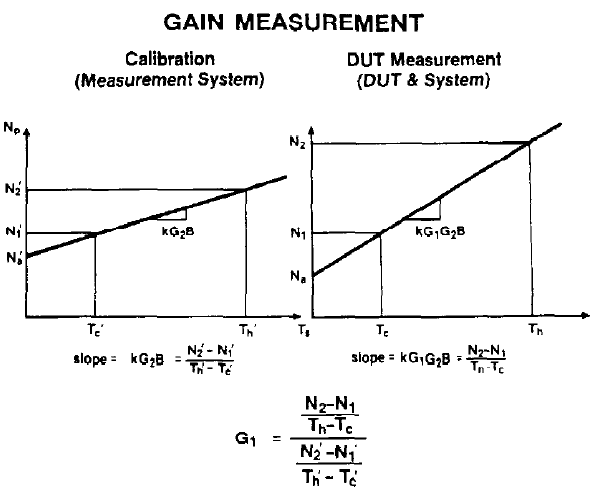Calculation the Second Stage Noise Contribution (Continued)

To separate the first and second stage noise contributions, we need to determine the gain of the first stage. Fortunately, this information is included in the slope of the noise power vs source temperature graphs. Specifically, the difference in the slopes for the two noise power vs source temperature graphs shown is the gain of the DUT. Thus we can determine the gain and noise figure for the DUT by first measuring the noise figure and gain of the test system (through system calibration), next measuring the noise figure and gain of the DUT and the test system together, and then calculating the DUT's gain using the equation shown and calculating the noise figure using the equation on the previous slide.

Excess Noise Ratio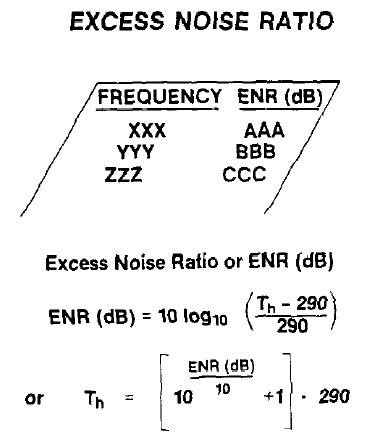Definition

Electronic noise sources used for Y factor noise figure measurements are furnished with a calibration report which characterizes the output noise power of the noise source in terms of excess noise ratio vs frequency. The mathematical relationship between excess noise ratio and the hot noise temperature, Th, of the electronic noise source is shown above. Tc, the cold noise temperature of the electronic load and the temperature of the noise source’s output pad is assumed to be 290 Kelvin. This data is used to enter the calibration factors for the Noise source once it is returned from it's annual trip being measured by a Calibration Standards Lab. This will be covered in later sections of the seminar.

Noise Figure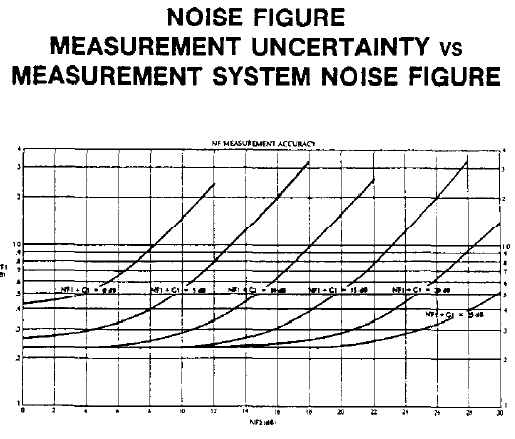As you can see from the plot, the accuracy of the test system is a function of the DUTs noise figure & gain, and the noise figure of the measurement system. The lower the measurement system’s noise figure, the lower the measurement uncertainties. The noise figure of the Cassini Test System is approximately 8 dB. Please note, the curves are offset from 0 dB by the uncertainty of the NIST traceable standards currently available.

The Cassini ATE Systems provide high speed, high accuracy noise figure measurements, performing Y factor noise figure measurements in less than 75 msec typically and with better than 0.125 dBrms repeatability. Even better repeatability may be obtained by performing more measurements and averaging.

Single or Double Side Band Noise Figure?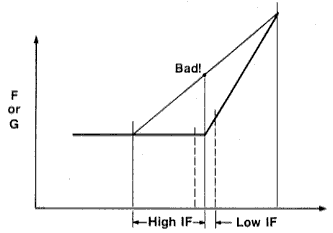How RI measures SSB Noise Figure

The Cassini and RI 7100A have narrow filters in their IF system, so it always makes a Single Side Band Noise Figure measurement and corrects the data in software to match what a typical Double Side Band measurement instrument would report. This is the same as bandpass filtering on a typical DSB Noise Figure meter. A DSB measurement is like an average of the independent Side Band readings, as shown above. If you are measuring an Image reject mixer with internal filtering or a device that has a rapid change of noise figure or gain with frequency, then the normal Noise Figure measurement can get confused as shown. The RI system has a special SSB Mixer Noise Figure button that takes this into account and reports the correct SSB noise figure directly.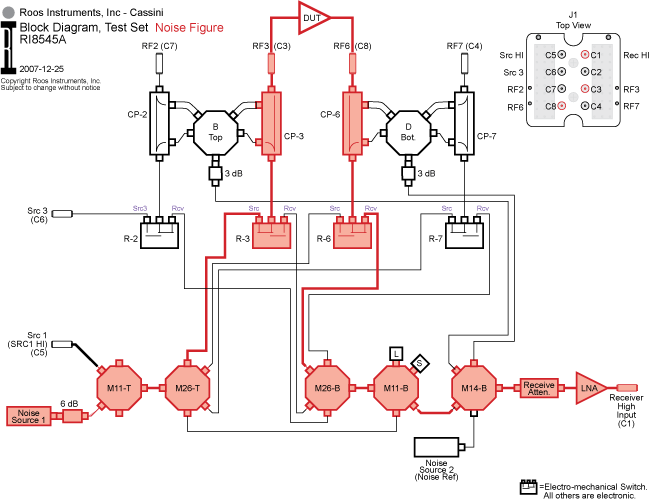Test Set Configuration for Noise Figure
The Test Set diagram shown is a typical noise figure measurement configuration. The noise source is switched into the port RF3 source path in place of Source1. The noise source is turned on and off and the noise energy is routed through the Test Set to the device-under-test’s (DUTs) RF input connected to port RF3. To measure the noise figure of the DUT shown. the system needs to measure the noise power of the transmitted signal into port RF6 from the DUT. The transmitted signal is routed (as shown above) through the "thru path" of the port RF6's (in this example) directional coupler, through the port direction low loss mechanical switch, three electronic switches and a RF preamplifier to the single channel System Receiver (port Rec HI at C1) for signal processing.Measurement Configuration for Noise Figure Measurements
The measurement process for Noise Figure by the System Receiver utilizes a True RMS Noise Power detector similar to the type used in industry standard Noise Figure meters, and the same Hot / Cold Noise technique. The system Lo frequency will be tuned for what the Source 1 stimulus frequency is set for and the RF signal from Source 1 will be turned off (-20 to -40 dB lower than set), the hot noise signal (with the Test Head Noise source turned on) from the DUT will be downconverted to a 21.4 MHz IF, The IF signal is conditioned (attenuated, filtered or amplified) and sent to the True RMS Noise Power detector. The resulting signal is sampled by high speed sample & hold circuits and the high accuracy A to D converter digitizes the sampled RMS power and sends the digitized data over the RIFL bus to the System Computer for processing. This process will be repeated for the cold noise signal (with the Test Head Noise source turned off ) and then the System Software will calculate the Noise Figure and report that value in dB. This process happens very fast, typically under 70 msec.

The Cassini System Noise Figure is about 8 dB, so a DUT with some gain is required to make good Noise Figure measurements. Since this is a second stage effect, (which we discussed earlier) a typical device with 8 -10 dB of gain can be measured to below 1 dB for Noise Figure measurements.

https://roos.com/docs/TDOO-5YFUFG
ROOS INSTRUMENTS CONFIDENTIAL AND PROPRIETARY